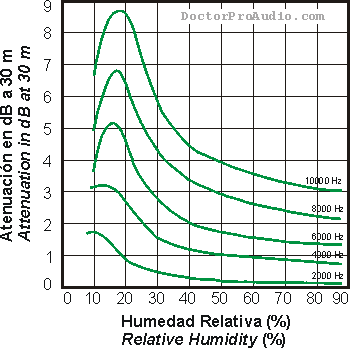Deutsche VersionDamping of Air at High Frequencies  (Dissipation)

 Calculation: Absorption of sound by the atmosphere depending on the damping as a function of frequency, temperature, and humidity Acoustics - Attenuation of sound during propagation outdoors - ISO 9613-1:1993 - No ultrasound   "Damping (decreasing) of sound with distance" is really a different fact. Don't mix it up with the damping (attenuation) of high frequencies of air.   How does distance affect sound? No frequency dependance. How do high frequencies affect sound? Frequency dependance.

 Temperature of air °C   °F Relative humidity % Frequency Hz ↓ Atmospheric absorption dB/m dB/100 m   dB/100 ft
 Fill the known values in the three enter boxes and press the calculate bar to calculate the air absorption. This is the added (!) air damping (dissipation of air), because this value must be added to the usual distance damping of audible sound after the 1/r law.

 Sorry, the above program does not work with "Google Chrome". Why this is the case, is unclear. It is the first program that does not work. Mozilla Firefox and Internet Explorer is OK.

 How does the "distance from the sound source" affect the sound? The sound level changes with distance, however, there is no frequency dependence. How does "the air attenuation at high frequencies" affect the sound? The sound level changes with distance, however, there is a strong frequency dependence.

 We have the usual "not frequency-dependent damping (decrease) of sound with distance" (distance attenuation) and the "frequency-dependent attenuation of the air at high frequencies" (dissipation). Both cases are to be considered separately and must therefore be calculated separately.

 The distance-dependent and frequency-dependent air absorption can be calculated, which is known as an additional (!) air damping or dissipation of the air, however, then the value of the calculated normal distance attenuation of the audible sound must be added after the 1/r law.

 Acoustics - Attenuation of sound during propagation outdoors - Calculation of the absorption of sound by the atmosphere ISO 9613-1:1993 specifies an analytical method of calculating the attenuation of sound as a result of atmospheric absorption for a variety of meteorological conditions. For pure-tone sounds, attenuation due to atmospheric absorption is specified in terms of an attenuation coefficient as a function of the frequency of the sound from 50 Hz to 10 kHz (no ultrasound), the temperature (−20 °C to +50 °C), the relative humidity (10 % to 100 %) and pressure (101325 Pa = 101.325 kPa) of the air. Formulae are also given for wider ranges and for other than pure tones − only audible sound 20 Hz to 20000 Hz.   Subsonic noise 0 Hz − 20 Hz, audible sound 20 Hz − 20 kHz, ultrasound > 20 kHz   It is often asked what formula stands for the calculation of the damping of air: Formula for calculating the air damping up to 10 kHz

 Frequency dependent attenuation of air (dB) in 30 m distance at different humidity (percent)How does the sound decrease with increasing distance? Calculation of distance damping:
Changing of the sound level Δ L with the distance r

 This air absorption is valid for the direct field D (free field), an outside location without contributing reflections. When calculating the additional high frequency attenuation of speaker signals in a living room caused by air absorption this formula (method) cannot to be used. Due to the additive reflections in the room field R (diffuse field of a room) the law of frequency dependent air damping does not apply. The changing of atmospheric pressure does not change the sound of musical instruments in a concert hall or in a room, because thus the speed of sound does not change; see: http://www.sengpielaudio.com/calculator-speedsound.htm

Composition of air

 Constant configuration (Values stay equal over time and place) Nitrogen (N2) 78.08 % Oxygen (O2) 20.95 % Argon (Ar) 0.93 % Neon, helium, krypton 0.0001 % Variable configuration (Values change over time and place) Carbon dioxide (CO2) 0.039 % Water vapor (H2O) 0 to 4 % Methane (CH4) traces Sulfur dioxide (SO2) traces Ozone (O3) traces Nitrogen oxides (NO, NO2) traces

 Forget really the dBA when measuring the damping of air. Words to bright minds: Always wonder what a manufacturer is hiding when they use A-weighting. *)